Next Article in Journal
Quantum Limits to the Second Law
Next Article in Special Issue
An Elementary Derivation of The Black Hole Entropy in Any Dimension
Previous Article in Journal
Entropy - Some Cosmological Questions Answered by Model of Expansive Nondecelerative Universe
Previous Article in Special Issue
Information Seen as Part of the Development of Living Intelligence: the Five-Leveled Cybersemiotic Framework for FIS

Entropy 2002, 4(6), 168-182; https://doi.org/10.3390/e4060168

Article
Entropy of Black Holes: A Quantum Algebraic Approach
byA. Iorio 2,G. Lambiase 1 andG. Vitiello 1,*1
Dipartimento di Fisica "E.R. Caianiello" Universitá di Salerno, 84081 Baronissi (Sa), Italy
2
Center for Theoretical Physics, Massachusetts Institute of Technology -77, Massachusetts Avenue - Cambridge MA 02139 - 4307, USA
*
Author to whom correspondence should be addressed.
Received: 15 May 2002 / Accepted: 3 February 2003 / Published: 3 February 2003

## Abstract

:
In this paper we apply to a class of static and time-independent geometries the recently developed formalism of deformed algebras of quantum fields in curved backgrounds. In particular, we derive: i) some non-trivial features of the entanglement of the quantum vacuum, such as the robustness against interaction with the environment; ii) the thermal properties and the entropy of black holes for space-times with a unique event horizon, such as Schwarzschild, de Sitter and Rindler space-times.
Keywords:
Quantum Algebras; Theory of quantized fields; Quantum Field Theory in curved space-times

## 1 Introduction

The quantization of matter fields in curved space-times is, as widely believed, a preliminary step towards a more complete theory of quantum gravity . In this framework the most important result is the thermal evaporation of black holes, whose temperature T is related to surface gravity κ by the relation κT ∼ (GM )−1, where M is the mass of the black hole . Such a result has been obtained also for Rindler space-time [3, 4, 5, 6, 7], corresponding to an accelerating observer, with the difference that the surface gravity is replaced by the acceleration a of the observers (κa).
In a recent paper , we have shown that the quantization of a complex scalar field in a curved manifold can be carried out in the framework of quantum algebras. In this new setting, one can study the thermal properties of quantum field theory in curved space-times. In particular, as shown in , one can derive the functional relation between the entropy of black holes and the area of the event horizon. To be more specific, we have shown that a suitable deformation of the algebra hk(1) of the annihilation and creation operators of the scalar fields, initially quantized in Minkowski space, induces the canonical quantization of the same field in a static gravitational background. The basic result arising from our approach is that the parameter of deformation q is related to the physical parameters characterizing the gravitational field, hence the event horizon (for example, the acceleration in the case of Rindler space-time, or the Schwarzschild radius for the Schwarzschild geometry). As a consequence, q is related to the surface gravity which is proportional to the inverse of the event horizon. This occurs in geometries with a single event horizon.
The aim of this paper is to present some applications of the formalism developed in . Namely, we shall emphasize the robustness against interaction with the environment of the entanglement of the quantum vacuum, due to the non-unitarity of the mapping between the vacua in the flat and curved frames of reference. Furthermore, we shall derive the entropy of black holes for the Schwarzschild, de Sitter, and Rindler geometries, characterized by having a unique event horizon.
The paper is organized as follows. In Section 2 we recall the main features of the formalism proposed in . Section 3 is devoted to the analysis of the vacuum properties for the Minkwoskian and the generic observers. This analysis shows that the entangled structure of the vacuum is robust against interaction with the environment in the infinite volume limit. Besides, we calculate the black hole entropy for static and stationary geometries with a unique event horizon. Conclusions are drawn in Section 4. Some further thermal features are commented in the Appendix.

## 2 Deformed Algebra, Thermal Operators and Entropy

A complex massive scalar field φ(x), quantized in Minkowski space-time, can be expanded in terms of the complete set of modes {Uk(x)},where $k = ( k 1 , k → ) , k → = ( k 2 , k 3 )$. The Hamiltonian operator is then given by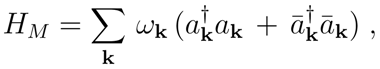where $ω k = k 1 2 + | k → | 2 + m 2$, and $a k , a k † ( a ¯ k , a ¯ k † )$ are the annihilation and creation operators, respectively, for particles (antiparticles). They act on the Fock space $H$, whose Minkowski vacuum is defined by $a k | 0 M > = a ¯ k | 0 M = 0 , ∀ k$. The operators entering the standard expansion (1) satisfy the usual canonical commutation relations (CCRs) $[ a k , a ¯ k ′ † ] = δ k k ′$ and so on. Algebraically speaking, the CCRs identify an infinite number of Weyl-Heisenberg algebras hk(1), one for each momentum k. Within these algebras we want to use the operation of co-product, which will enable us to deal with the partition of the Minkowski space-time into two sectors (±). This is the preliminary step towards the introduction of the unique event horizon. The coproduct of the operators ak and $a ¯ k$ is defined asThe operators $a k ( σ )$ and $a ¯ k ( σ )$, σ = ±, satisfy the CCRsand similarly for $a ¯ k ( σ )$. They act on the full Hilbert space, i.e. $H ⊗ H$, where the ground state (vacuum) is defined as $| 0 M > ⊗ | 0 M >$. For brevity we shall indicate $| 0 M > ⊗ | 0 M >$ with $| 0 M >$, and $H ⊗ H$ with $H$. The q-deformation of the coproduct (3) iswhere q = q(p). In what follows we shall consider the case of real deformation parameter q. More generally, q could depend on a momentum p which may or may not coincide with k. Furthermore, q may depend also on other parameters: q = q(phys, p) (where “phys” stands for the proper physical quantity [8, 9]). We also require that that $q ( p ) = q ( p ˜ )$, (here $p ≡ ( Ω , p → )$, $p ˜ ≡ ( Ω , − p → )$, Ω > 0, and $p → = ( k 2 , k 3 )$, i.e. q-deformation plays the same role on particles and antiparticles. Furthermore we shall make use of the standard relation $q ( p ) = e 2 ϵ ( p )$.
As explained in Ref. , by using the deformed coproducts (5), one can construct new operators acting in an Hilbert space with a non-flat (non-Minkowskian) space-time as a support. To preserve the canonical algebra we use a complete orthonormal set of functions {F(k, p)} to introduce the smeared operatorsThe q-deformed coproduct of d and $d ¯$ is given by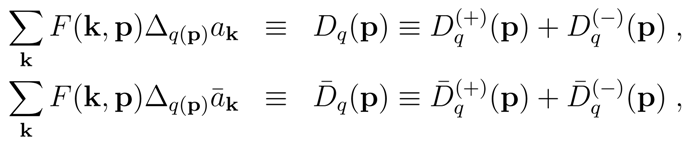respectively, where the following short-hand notation has been usedBy using (8) we can simply take suitable linear combinations to obtain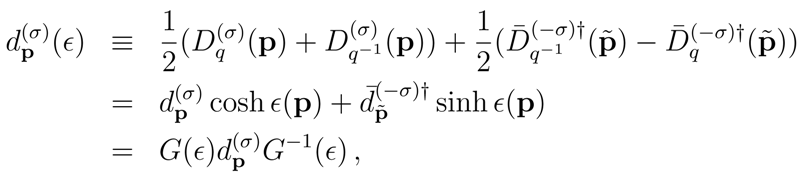and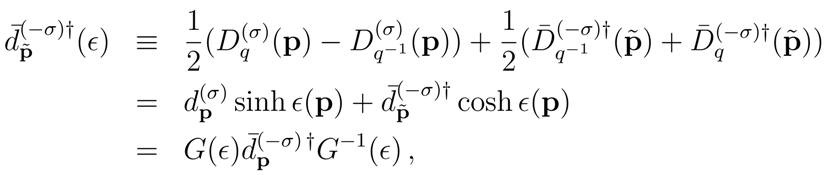Here G(ϵ) ≡ exp g(ϵ), whereG(ϵ) is the generator of the Bogoliubov transformations (9) and is a unitary operator at finite volume: $G − 1 ( ϵ ) = G ( − ϵ ) = G † ( ϵ )$.
Eqs. (9) and (10) relate vectors of $H$ to vectors of another Hilbert space $H ϵ$ labelled by ϵ. The relation between these spaces is established by the generator $G ( ϵ ) : H → H ϵ$. For the vacuum state one has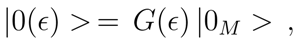where $| 0 ( ϵ ) >$ is the vacuum state of the Hilbert space $H ϵ$ annihilated by the new operators $( d p ( σ ) ( ϵ ) , d ¯ p ˜ ( σ ) ( ϵ ) )$. Note that even though ϵ = ϵ(phys, p), the vacuum |0(ϵ) > does not depend on p, but only on the physical parameter, as follows from Eq. (11). Furthermore |0(ϵ) > appears to be a SU (1, 1) generalized coherent state  of Cooper-like pairs (Bose-Einstein condensate). Of course similar considerations hold also for the state $| 0 M >$ by reversing G(ϵ) in Eq. (20). One can also see that the Hilbert spaces $H$, $H ϵ$, and $H ϵ ′$, $∀ ϵ ≠ ϵ ′$, become unitarily inequivalent in the infinite volume limit (V-limit).
We now observe that the physical meaning of having two distinct momenta k and p for states in the Hilbert spaces $H$ and $H ϵ$, respectively, is the occurrence of two different reference frames: the M-frame (Minkowski) and the new frame, which we shall call Mϵ-frame. To explore the physics in the Mϵ–frame, one has to construct a diagonal operator which plays the role of the Hamiltonian. To this end we exploit the deformation of the algebra above introduced (see Eq. (5)). One starts by considering the (1, 0)–component of the generator of the Lorentz transformations defined as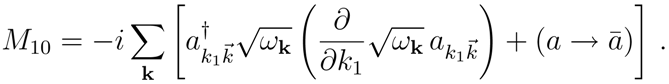By deforming the coproduct of M10, one can write the Hamiltonian aswhere the operators $d p ( σ ) ( ϵ )$ and $d ¯ p ˜ ( σ ) ( ϵ )$ are given in (9). Notice that $H ϵ$ acts on states defined in the new Hilbert space $H ϵ$ and has as space–time the new frame $M ϵ$.
Turning now our attention to $| 0 M >$, from Eq. (9) one gets that the number of modes of the type $d p ( σ ) ( ϵ )$ in $| 0 M >$ is given bywhere $N d ( ϵ ) ( σ ) = d p ( σ ) † ( ϵ ) d p ( σ ) ( ϵ )$. An analogous formula holds for the modes of type $d ¯ p ˜ ( σ ) ( ϵ )$. Eq. (15) makes clear the condensate structure of $| 0 M >$. While one can work indifferently in one of the two sectors, σ = + or σ = −, we shall use σ = +.
The entropy $S ≡ S ( + ) ( ϵ )$ of the Minkowskian vacuum as felt by the observer in the Mϵ-frame, is given by .The entropy operator (16) agrees with the definition of von Neumann entropy , where $N$ is the number of microscopic states. For the reader convenience, in the Appendix we shall comment on further thermal properties. In particular we observe that, under extremal conditions, the number of particles $N d ( ϵ ) ( + )$ in the Mϵ-frame computed in the Minkowski vacuum $| 0 M >$ gives a Bose-Einstein distribution. In the regime of thermal equilibrium, the induced partition of the Mϵ space–time into two sectors, σ = + and σ = −, indicates the emergence of an event horizon, namely the emergence of a gravitational field. All that is encoded in the condensate structure of |OM >. As can be seen from Eqs. (4) and (5) in the Appendix, a constant temperature T = β−1 implies that the Mϵ-frame is static and time-independent.
We remark that one of the advantages of the approach outlined above relies on the fact that the CCRs in the Mϵ–frame have not to be imposed by hands, as usually done in QFT in curved space–time, but they are naturally recovered via q-deformation . The physical parameter characterizing the background geometry is related to the q-deformation parameter.

## 3 Applications to Schwarzschild, de Sitter and Rindler Space–Times

Our aim now is to apply the previous results to some specific geometries. First we derive the functional relation between the event horizon and the surface gravity in geometries with a unique event horizon. Let us consider a space-time with spherical symmetry whose line element is of the formThe surface gravity κ is defined as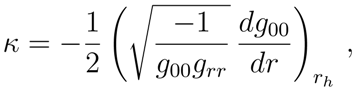where rh is the value for which $g 00 ( r h ) = 0$. Let us consider the usual case in which the components of the background geometry are such that $g 00 = g r r − 1$ and that $g 00$ has the formwhere n can be positive or negative, and α and γ are numerical factors. From $g 00 ( r h ) = 0$ it follows $r h = α / γ n$. With a simple calculation, one immediately derives that the surface gravity isConsequences of this result will be studied in the following Subsections in the framework of Schwarzschild, de Sitter and Rindler geometries. Nonetheless, before moving to those applications, we want to present other important results of our treatment of the vacuum entanglement. Note that these features are common to all the above mentioned geometries.

#### 3.1 Vacuum Structure and Entanglement

As we have noted, the relation between the spaces $H$ and $H ϵ$ is established by the generator $G ( ϵ ) : H → H ϵ$, or by its inverse $G − 1 ( ϵ ) : H ϵ → H$. Thus the Minkowskian vacuum is expressed in terms of the generic ϵ-vacuum as followsThis relation holds only at finite degrees of freedom, i.e. finite volume. Note that G(ϵ) is an element of2 SU(1, 1) × SU(1, 1). Of course the same structure arises by writing G−1(ϵ) in terms of the d(ϵ)s, all one has to do is to replace dd(ϵ) in the Eq. (11). Thus by using the Gaussian decomposition, the Minkowski vacuum can be expressed as a SU(1, 1) × SU(1, 1) generalized coherent state  of Cooper-like pairswhere Z = ∏p cosh2 ϵ(p).
In the continuum limit in the space of momenta, i.e. in the infinite-volume limit, the number of degrees of freedom becomes uncountable infinite, hence we have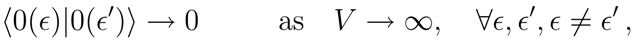where V is the volume of the whole (D − 1)-dimensional space. This means that the Hilbert spaces $H$ and $H ϵ$ become unitarily inequivalent in the continuum limit. In this limit ϵ, related to the deformation parameter by the relation $q = e 2 ϵ$, labels the set ${ H ϵ , ∀ ϵ }$ of the infinitely many unitarily inequivalent representations of the CCRs
Let us now discuss the entanglement of the vacuum $| 0 M 〉$ in (21), that we rewrite in the following convenient formwhere, we denote by $| n p ( σ ) , m ¯ p ˜ ( σ ) 〉$ a state of n particles and m antiparticles in whichever sector (σ). Note that for the generic nth term, the state $| n p ( σ ) , 0 ¯ 〉 ≡ | 1 p 1 ( σ ) , … , 1 p n ( σ ) , 0 ¯ 〉$, and similarly for antiparticles.
By introducing a well known notation, ↑ for a particle, and ↓ for an antiparticle, the two-particle state in (24) can be written aswhich is an entangled state of particle and antiparticle living in the two causally disconnected regions (±). The generic nth term in (24) shares exactly the same property as the two-particle state, but this time the ↑ describes a set of n particles, and ↓ a set of n anti-particles. The mechanism of the entanglement, dynamically induced by gravitational effects, takes place at all orders in the expansion, always by grouping particles and antiparticles into two sets. Thus the whole vacuum $| 0 M 〉$ is an infinite superposition of entangled states3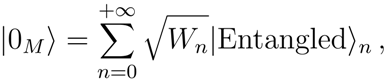where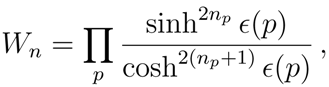withThe coefficients $W n$ of the expansion in Eq. (24) appear also in the discussion about the entropy of the black hole on which we shall concentrate in the following Sections. Details of calculations can be found in Appendix A.
Of course, the probability of having entanglement of two sets of n particles and n antiparticles is Wn. At finite volume, being Wn a decreasing monotonic function of n, the entanglement is suppressed for large n. It appears then, that only a finite number of entangled terms in the expansion (26) is relevant. Nonetheless this is only true at finite volume (the quantum mechanical limit), while the interesting case occurs in the infinite volume limit, which one has to perform in a quantum field theoretical setting.
The entanglement is generated by G(ϵ), where the scalar field modes in one sector (σ) are coupled to the modes in the other sector (−σ) via the parameter ϵ(p). We remark that ϵ(p) actually describes the ”environment”, namely ϵ(p) takes into account the effects of the background gravitational field . Surprisingly in the present formulation the origin of the entanglement is the environment, in contrast with the usual quantum mechanical view, which attributes to the environment the loss of the entanglement. In the present treatment such an origin for the entanglement makes it quite robust.
One further reason for the robustness is that this entanglement is realized in the limit to the infinite volume once and for all, since then there is no unitary evolution to disentangle the vacuum: at infinite volume one cannot ”unknot the knots”. Such a non-unitarity is only realized when all the terms in the series (26) are summed up, which indeed happens in the V → ∞ limit. In a future work we will comment with more details on the specific topic of the entanglement.

#### 3.2 The Schwarzschild Geometry

We shall start with the Schwarzschild geometry. In polar coordinates, the line element of this space–time is (in natural units)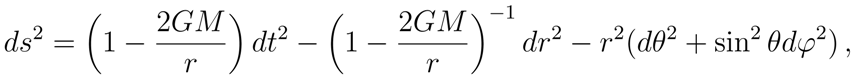α = 1, γ = 2GM and n = −1 in (19). The event horizon is given by rh = 2GM. According to our discussion (see Section II and the Appendix), the parameter β is related to the surface gravity, so that we can impose the identification β ~ rh, or T ~ (GM)−1, which is the Bekenstein-Hawking temperature defined in the Introduction. The q-deformation parameter is related to β (see Appendix) hence to the Schwarzschild radius rS = 2GMrh, which characterizes the geometrical structure underlying the Mϵ-frame.
Let us now analyze the entropy operator. At the origin of the entropy (16) there are the vacuum fluctuations of quantum states, which have a thermal character for different observers related to the Minkowski observer through a diffeomorphism. In a curved space-time the momentum concept can be defined only locally. Keeping this in mind, it is convenient to make calculations in the continuum limit,We shall use the relationwhere $b ( + ) ( p → )$ is a wave-packet $( ∫ | b ( + ) ( p → ) | 2 d 2 p < ∞ )$, similarly for $d ¯ p ˜ ( + ) † ( ϵ )$.
The expectation value on the vacuum $| 0 M >$ of the entropy operator defined in Eq. (16), gives the entropy density, $s ( + ) ( ϵ ) ≡ < S ( + ) ( ϵ ) > M / V$, henceup to a factor of dimensions [lenght]−2. The integration in Eq. (30), see also Eq. (4) in the Appendix, yieldsFrom Eq. (31) immediately follows that the entropy $< S ( + ) ( ϵ ) > M$ of a spherical shell of radius δ is4, again up to a factor of dimensions [lenght]−2,namely the entropy is proportional to the horizon area $A$ of the black holes [2, 15].

#### 3.3 The de-Sitter Space–Time

Another interesting static geometry is the de Sitter one characterized by the line element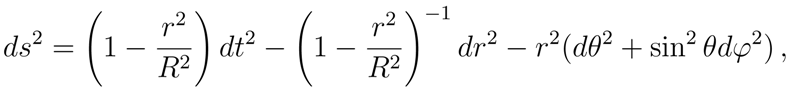which clearly exhibits a horizon at rh = R. Here R is the radius of the four-dimensional hyperboloidembedded in a five-dimensional flat space-time. The comparison with (19) gives α = 1, γ = 1/R2 and n = 2. By using (18) it follows that $κ = R − 1 = r h − 1$. For the above argument, β ~ R. Thus the entropy s(+) turns out to be
$s ( + ) ~ β − 1 ~ R − 1$
and the total entropy is given by (see (30)-(32))
$< S ( + ) ( ϵ ) > M ~ R 3 s ( + ) ~ R 2 ~ A$
and again the proportionality between entropy and area of the horizon is recovered.

#### 3.4 The Rindler Geometry

As further example, we shall now analyze a geometry in which again only one horizon is present but $g 00 ≠ g r r − 1$. This is the case of Rindler space-time. It is described by the line element (see for example )which reduces to Minkowski space-time lettingThis metric covers a portion of Minkowski space-time with x > |t|. The boundary planes are determine by x ± t = 0 .
From Eq. (18) we get κ = a = 1/β. The volume V is given bywhere 𝒜 = ∫ dyRdzR is the area of the surface of constant x and it has been evaluated at ξ = 0. Following the same calculations as for the Schwarzschild case, one infers that the entropy density turns out to be
$s ( + ) ~ β − 1 ~ a$
so that the entropy is
$< S ( + ) ( ϵ ) > M ~ A a − 1 s ( + ) ~ A$
which agrees with the result of Ref. .
It is worth noting that in the case of Rindler space-time, results are formally equivalent to the Schwarzschild geometry since the surface gravity of the black holes is the gravitational acceleration at radius r measured at the infinity.

## 4 Conclusions

In this paper we exploited a model developed in a previous paper , where it was shown how the q-deformation of the canonical algebra of a complex scalar field quantized in the Minkowski space–time reproduces some of the typical structures of a quantized field in a space–time with a unique horizon. The deformation parameter q depends on physical quantities related to the geometrical properties characterizing the background. The parameter q(phys) → 1, as the physical parameters vanish, and the curved space-time reduces to a locally flat space-time.
We are then led to conclude that: i) quantum deformations can be described in terms of gravitational field effects; ii) the origin of the vacuum entanglement is the environment (contrarily to the common view which attributes to the environment the loss of the entanglement); iii) this entanglement is realized in the limit to the infinite volume once and for all, since then there is no unitary evolution to disentangle the vacuum. For the last two reasons the entanglement in this context is quite robust.
On the other hand, we have also derived the relationship between the entropy and the area of event horizons for Schwarzschild, de Sitter and Rindler space-times. The results obtained here, as well as in , hold for geometries in which the horizon separates the space-time in two regions. It will be certainly interesting to extend our analysis to the case of geometries with more than one horizon, where the space-time is made of more than two regions. For instance, in the Kerr and Reissner-Nordstrom geometries one has two horizons, r+ and r, to which correspond two distinct surfaces gravity, k+ and k, as well as two distinct temperatures, T+ and T (k± ~ T±)
The key point in handling such geometries in our approach is to find a suitable deformation of the co-product. This implies a more careful analysis in deriving, from the deformed algebra, the Bogoliubov transformations (9) that are at the core of the thermal properties inferred in . This work is currently under investigation, as well as the extension to the fermionic fields.

## Acknowledgments

A.I. and G.V. acknowledge the financial support of the European Science Foundation, Network COSLAB. A.I. has been partially supported by the Fellowship “Theoretical Physics of the Fundamental Interactions”, University of Rome Tor Vergata. The authors thank the referees for constructive comments and suggestions.

## Appendix: Thermal Properties and Free Energy

The generator of the Bogoliubov transformations g(ϵ), in Eq. (11), is one of the generators of SU(1, 1), as can be easily seen by Eq. (9). Thus one can invert Eq. (20), and use the Gaussian decomposition for SU(1, 1) (see, for instance, Ref.), to express the Minkowski vacuum aswhere Z = ∏p cosh2 ϵ(p). Morover, < 0(ϵ)|0(ϵ) > = 1, ∀ϵ, and in the infinite-volume limit, we have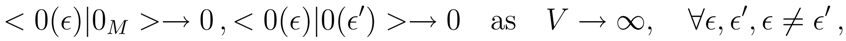i.e. the Hilbert spaces $H$, $H ϵ$ and $H ϵ ′$, $∀ ϵ ≠ ϵ ′$, become unitarily inequivalent in the infinite volume limit (V -limit).
By knowing the Hamiltonian and the entropy, Eqs. (14) and (16) respectively, one can define the free-energy as [19, 13, 20]where β = T−1. Looking for values of ϵ(p) making $F ( + ) ( ϵ )$ stationary, one obtains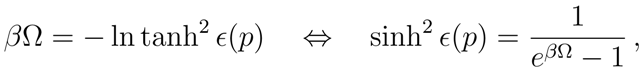from which, by using Eq. (15), follows thatand similarly for $N d ¯ ( ϵ ) ( + )$. We stress here that Eq. (5) is a direct consequence of the interplay between the doubling (d(+)(ϵ) and d(−)(ϵ), and similarly for the antiparticles) built in the coproduct, and the deformation of the latter.
Summarizing : i) β is related to the event horizon, namely to the surface gravity; ii) being β constant in time, the Mϵ frame is static and time-independent, in agreement with the fact that we deal with systems at thermal equilibrium; iii) the gravitational field itself vanishes as the deformation parameter ϵ → 0.

## References

1. Birrel, N.D.; Davies, P.C.W. Quantum Fields in Curved Space; Cambridge University Press: Cambridge, 1982. [Google Scholar]
2. Hawking, S.W. Commun. Math. Phys. 1975, 43, 199.
3. Davies, P.C.W. J. of Phys. 1975, A8, 609.
4. Unruh, W.G. Phys. Rev. 1976, D14, 870.
5. Takagi, S. Progress of Theor. Phys. Suppl. 1986, 88.
6. Wald, R.M. Quantum Field Theory in Curved Spacetime and Black Hole Thermodynamics; University of Chicago Press, 1994. [Google Scholar]
7. Fulling, S.A.; Ruijsenaars, S.N.M. Phys. Rep. 1987, 152, 137, and references therein.
8. Iorio, A.; Lambiase, G.; Vitiello, G. Ann. Phys. 2001, 294, 234.
9. De Martino, S.; De Siena, S.; Vitiello, G. Int. J. Mod. Phys. 1996, B10, 1615. Celeghini, E.; De Martino, S.; De Siena, S.; Iorio, A.; Rasetti, M.; Vitiello, G. Phys. Lett. 1998, A244, 455.
10. Perelomov, A. Generalized Coherent States and Their Applications; Springer: Berlin, Heidelberg, 1986. [Google Scholar]
11. Itzykson, C.; Zuber, J.B. Quantum Field Theory; McGraw–Hill, 1980. [Google Scholar]
12. Jing, J. Il Nuovo Comento 1998, 113B, 1075.
13. Umezawa, H.; Matsumoto, H.; Tachiki, M. Thermo Field Dynamics and Condensed States; North Holland, 1982. [Google Scholar]
14. Gradshteyn, I.S.; Ryzhik, I.M. Table of Integrals, Series and Products, Fifth Edition ed; Academic Press; San Diego, CA, 1982. [Google Scholar]
15. Bekenstein, J.D. Phys. Rev. 1974, D9, 3292. Wald, R.M. Black Holes and Relativistic Stars; Wald, R.M., Ed.; University of Chicago Press, 1999. [Google Scholar] Mitra, P. Entropy of Extremal Black Holes; hep-th/9704201.
16. Polarski, P. Phys. Rev. 1990, D41, 442.
17. Rindler, W. Essential Relativity; Springer: Berlin, 1977. [Google Scholar]
18. Laflamme, R. Phys. Lett. 1987, B196, 449.
19. Takahashi, Y.; Umezawa, H. Int. J. Mod. Phys. 1996, B10, 1755. (reprinted from Collect. Phenomen. 1975, B10, 2.)
20. Celeghini, E.; Rasetti, M.; Vitiello, G. Ann. Phys. 1992, 215, 156.
• 1Now at: Center for Theoretical Physics, Massachusetts Institute of Technology -77, Massachusetts Avenue - Cambridge MA 02139 - 4307, USA. E-mail: [email protected]
• 2To be more precise, for each mode, we have the direct product of two two-boson realizations of SU(1, 1): $[ T + i , T − i ] = − 2 T 0 i$, $[ T 0 i , T ± i ] = ± T ± i$, i = 1, 2, where [T1, T2] = 0, for all the generators T. This is seen by defining $T + 1 = d ( + ) † d ¯ ( − ) †$, $T − 1 = d ( + ) d ¯ ( − )$, $T 0 1 = 1 2 ( d ( + ) † d ( + ) + d ¯ ( − ) † d ¯ ( − ) )$, while $T + 2$, $T − 2$, $T 0 2$ are obtained by replacing (+) ↔ (−).
• 3A similar structure also arises in the temperature-dependent vacuum of Thermo-Field Dynamics .
• 4The proper volume $V$ of the shell is determined by the relation
where h = δ/rh << 1.